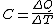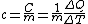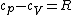# specific heat

Also found in: Dictionary, Thesaurus, Medical, Legal, Acronyms, Wikipedia.

## specific heat,

ratio of the heat capacityheat capacity
or thermal capacity,
ratio of the change in heat energy of a unit mass of a substance to the change in temperature of the substance; like its melting point or boiling point, the heat capacity is a characteristic of a substance.
of a substance to the heat capacity of a reference substance, usually water. Heat capacity is the amount of heatheat,
nonmechanical energy in transit, associated with differences in temperature between a system and its surroundings or between parts of the same system. Measures of Heat
needed to change the temperature of a unit mass 1°. The heat capacity of water is 1 calorie per gram per degree Celsius (1 cal/g-°C;) or 1 British thermal unit per pound per degree Fahrenheit (1 Btu/lb-°F;). Thus, the specific heat of some other substance relative to water will be numerically equal to its heat capacity; for this reason, "specific heat" is often used when the heat capacity actually is meant. Because the heat capacities of most substances vary with changes in temperature, the temperatures of both the specified substance and the reference substance must be known in order to give a precise value for the specific heat. The heat capacity of water at 15°C; is a frequently used value. Like specific gravity, specific heat is a dimensionless quantity, i.e., a pure number having no unit of measurement associated with it.

## Specific heat

A measure of the heat required to raise the temperature of a substance. When the heat ΔQ is added to a body of mass m, raising its temperature by ΔT, the ratio C given in Eq. (1) is defined as the heat capacity of the body. The quantity c defined in Eq. (2) is

(1)(2)called the specific heat capacity or specific heat. A commonly used unit for heat capacity is joule · kelvin-1 ( J · K-1); for specific heat capacity, the unit joule · gram-1 · K-1 ( J · g-1 · K-1) is often used. Joule should be preferred over the unit calorie = 4.18 J. As a unit of specific heat capacity, Btu · lb-1 · °F-1 = 4.21 J · g-1 · K-1 is also still in use in English-language engineering literature. If the heat capacity is referred to the amount of substance in the body, the molar heat capacity cm results, with the unit J · mol-1 · K-1.

If the volume of the body is kept constant as the energy ΔQ is added, the entire energy will go into raising its temperature. If, however, the body is kept at a constant pressure, it will change its volume, usually expanding as it is heated, thus converting some of the heat ΔQ into mechanical energy. Consequently, its temperature increase will be less than if the volume is kept constant. It is therefore necessary to distinguish between these two processes, which are identified with the subscripts V (constant volume) and p (constant pressure): CV, cV, and Cp, cp. For gases at low pressures, which obey the ideal gas law, the molar heat capacities differ by R, the molar gas constant, as given in Eq. (3), where R = 8.31 J · mol-1 · K-1; that is, the expanding gas heats up less.

(3)For solids, the difference between cpand cVis of the order of 1% of the specific heat capacities at room temperature. This small difference can often be ignored. See Heat capacity, Thermodynamic processes

## specific heat

[spə′sif·ik ′hēt]
(thermodynamics)
The ratio of the amount of heat required to raise a mass of material 1 degree in temperature to the amount of heat required to raise an equal mass of a reference substance, usually water, 1 degree in temperature; both measurements are made at a reference temperature, usually at constant pressure or constant volume.
The quantity of heat required to raise a unit mass of homogeneous material one degree in temperature in a specified way; it is assumed that during the process no phase or chemical change occurs.

## specific heat

The ratio of the quantity of heat required to raise the temperature of a given mass of any substance 1 degree to the quantity required to raise the temperature of an equal mass of water 1 degree.
References in periodicals archive ?
p] is the gaseous specific heat, [rho] is gaseous density, P[r.
After repeated testing, we felt the method accurately predicted the value of the specific heat of the plastics as reported by others.
The normal value of specific heat is the effective specific heat when the calcined petroleum coke porosity is 0.
Additionally, it was taken into account that cooling need in the office building was excessively high, for the cases with lower specific heat loss much higher than heating need, as can be seen in Table 2.
To apply this method for measuring the specific heat capacity of fuels in a heating process, several methodical difficulties had to be overcome by the researchers.
The specific heat has been measured in a Calvet microcalorimeter.
Differential thermal analysis device is one of the thermal identification and analysis devices, which is used for the determination of enthalpy changes and the value of specific heat capacity of materials at constant pressure in an exothermic or endothermic chemical reaction.
The various thermal properties such as thermal conductivity, diffusivity and specific heat of polyester/ natural fiber (banana/sisal) composites were investigated by Idicula et al.
The isobaric specific heat of a food material generally decreases with increases in pressure.

Site: Follow: Share:
Open / Close